[Top] [Next] [Prev]

## B.2 Logic Gates from Resistors, Diodes, and Transistors

In this subsection, we investigate how to construct logic functions from fundamental electrical objects like resistors, diodes, and transistors.

### B.2.1 Voltage Dividers

When a voltage spans two resistors in series, the voltage measured at the point between the resistors is divided in proportion to the ratio of the individual resistors and the sum of the resistances. As an example, see Figure B.4`(`a`)`.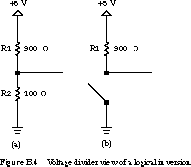The voltage across R1 is given by, where V is the voltage across the series resistors, R1 is the resistance between the power supply and the output node, and R2 is the resistance between the ground and the power supply. R1 is often called the load or pull-up resistor, while R2 is the pull-down resistor.

In the example, R1 `=` 900 ohms, R2 `=` 100 ohms, and V `=` 5 volts. The voltage drop across R1 is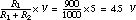Thus, the voltage measured between the output node and ground would be 0.5 V. Alternatively, we could have arrived at this directly by calculating the voltage across R2, namely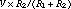. By appropriately sizing the relative resistances, we can choose any desired output -voltage.

Now suppose that R2 is a variable resistor with two basic settings: low resistance or very high resistance. When the pull-down resistor has a sufficiently high resistance, it behaves like an open circuit, as shown in Figure B.4`(`b`)`. The output node reaches 5 V.

An electrical device that can switch between low resistance and very high resistance is called a transistor, as we will see in Sections B.3 and B.4. A transistor is a three-terminal device that establishes a low-resistance path between two terminals when a high voltage is placed on the third terminal. When a low voltage is placed on this control terminal, the remaining two terminals are separated by a high resistance. If R2 is replaced by a transistor, it's easy to see that we obtain an inverter. When the input voltage is high, the output voltage is low. When it is low, the output voltage is high.

But before we can examine transistors in more detail, we need to take a look at a simpler, two-terminal device: the diode.

### B.2.2 Diode Logic

A diode is a two-terminal electrical device that allows current to flow in one direction but not the other. It is like a pipe with an internal valve that allows water to flow freely in one direction but shuts down if the water tries to flow backward. The schematic diagram for a diode is shown in Figure B.5.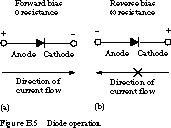The diode's two terminals are called the anode and cathode. In the diode symbol, the arrow points from the anode `(`flat part of triangle`)` toward the cathode `(`point of the triangle`)`.

The device operates by allowing current to flow from anode to cathode, basically in the direction of the triangle. Recall that current is defined to flow from the more positive voltage toward the more negative voltage `(`electrons flow in the opposite direction`)`. If the diode's anode is at a higher voltage than the cathode, the diode is said to be forward biased, its resistance is very low, and current flows. The diode is not a perfect conductor, so there is a small voltage drop, approximately 0.7 V, across it. If the anode is at a lower voltage than the cathode, the diode is reverse biased, its resistance is very high, and no current flows.

We can construct simple gates with nothing more than two or more diodes and a resistor. See Figure B.6.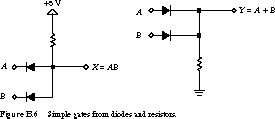At the left of the figure is a diode AND gate, and at the right a diode OR gate. Let's examine the AND gate first. If one of the inputs A or B is grounded, current flows through the diode and the output node X is at a low voltage. The only way to get a high output is by having both inputs high. This is clearly a logical AND function.

Now we turn to the OR gate. Whenever one or the other of the inputs A and B are high, current flows through the associated diode. This brings the output node Y to a high voltage. This circuit clearly implements a logical OR.

Unfortunately, it is difficult to cascade circuits of this kind into multiple levels of logic gates. The voltage drops across the diodes add up as they are cascaded in series, leading to significantly degraded voltage levels.

For example, suppose we wire up five diode-resistor AND gates in series. If a string of inputs are logic 0 and the series diodes are conducting, then the output from the final stage should be recognized as a logic 0 as well. But because each diode adds a 0.7-V drop, the measured output would actually be at 3.5 V. This is pretty far from any voltage that would be recognized as logic 0.

One solution is to increase the power supply voltage, redefining the range that is recognized as a logic 0 and logic 1. Of course, the higher the voltage, the higher the power consumed and the more heat the circuit generates. And no matter what you set the power supply to, there is still a limit to the number of logic levels that can be cascaded. This is hardly an adequate solution.

Also note that it is not possible to construct an inverter with only diodes and resistors. AND and OR functions by themselves are not a complete logic without NOT. Thus, there are some logic functions that cannot be implemented in diode-resistor logic. Fortunately, transistors solve all of these problems.

[Top] [Next] [Prev]